Publicité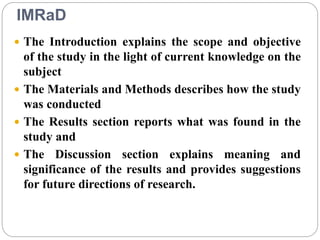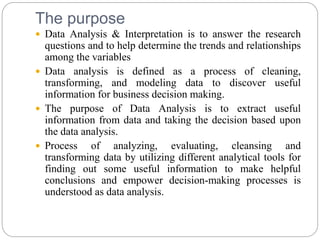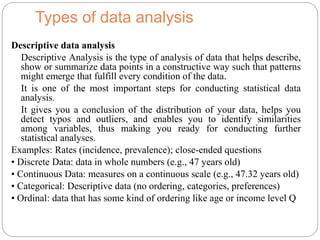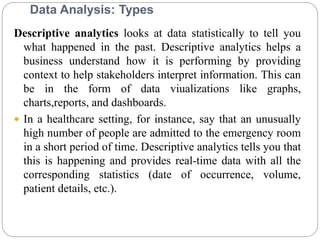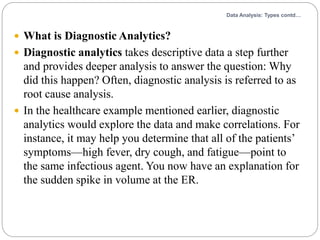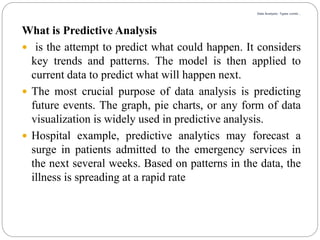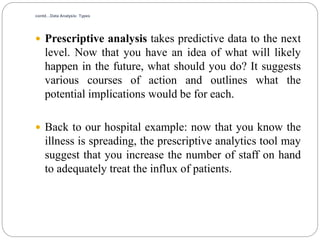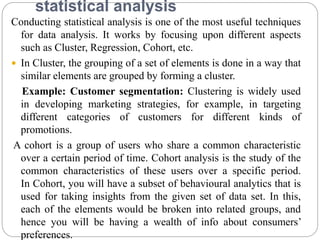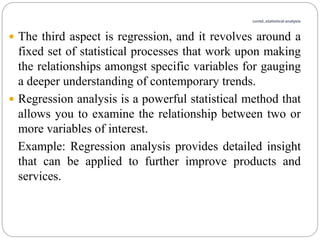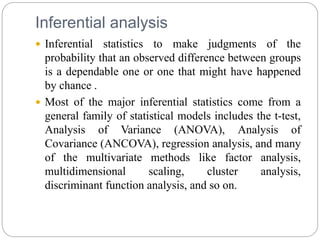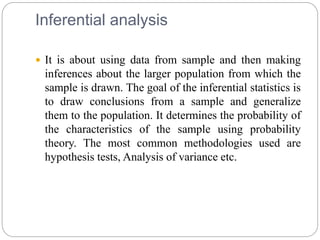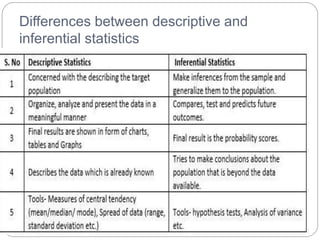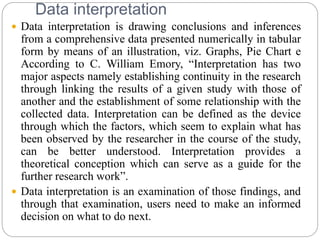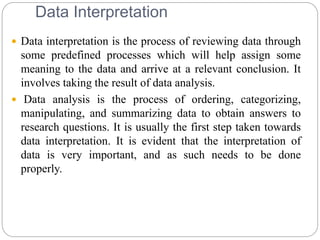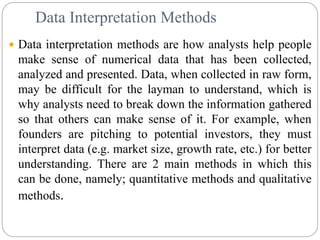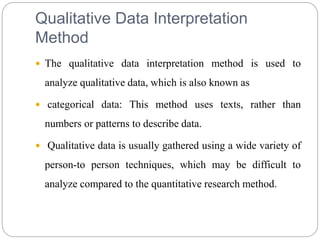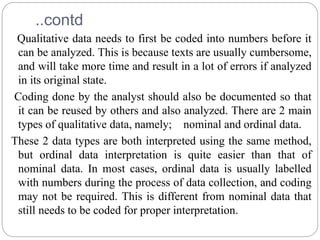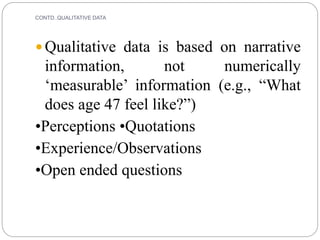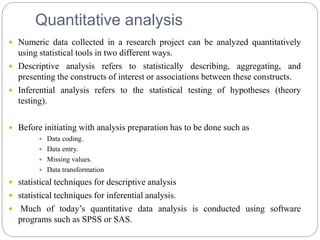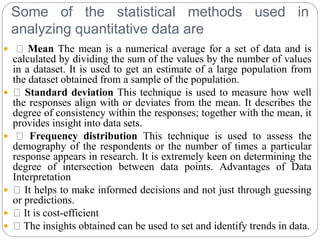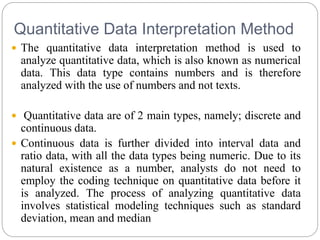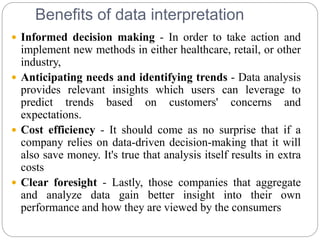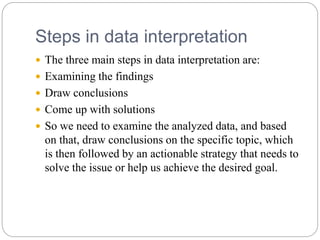1 sur 25
Publicité

### Data analysis aug-11

1. BY DR. Y. VINODHINI-PROFESSOR ANWARUL ULOOM COLLEGE OF BUSINESS MANAGEMENT, HYDERABAD DATA ANALYSIS AND INTERPRETATION
2. IMRaD  The Introduction explains the scope and objective of the study in the light of current knowledge on the subject  The Materials and Methods describes how the study was conducted  The Results section reports what was found in the study and  The Discussion section explains meaning and significance of the results and provides suggestions for future directions of research.
3. The purpose  Data Analysis & Interpretation is to answer the research questions and to help determine the trends and relationships among the variables  Data analysis is defined as a process of cleaning, transforming, and modeling data to discover useful information for business decision making.  The purpose of Data Analysis is to extract useful information from data and taking the decision based upon the data analysis.  Process of analyzing, evaluating, cleansing and transforming data by utilizing different analytical tools for finding out some useful information to make helpful conclusions and empower decision-making processes is understood as data analysis.
4. Types of data analysis Descriptive data analysis Descriptive Analysis is the type of analysis of data that helps describe, show or summarize data points in a constructive way such that patterns might emerge that fulfill every condition of the data. It is one of the most important steps for conducting statistical data analysis. It gives you a conclusion of the distribution of your data, helps you detect typos and outliers, and enables you to identify similarities among variables, thus making you ready for conducting further statistical analyses. Examples: Rates (incidence, prevalence); close-ended questions • Discrete Data: data in whole numbers (e.g., 47 years old) • Continuous Data: measures on a continuous scale (e.g., 47.32 years old) • Categorical: Descriptive data (no ordering, categories, preferences) • Ordinal: data that has some kind of ordering like age or income level Q
5. Data Analysis: Types Descriptive analytics looks at data statistically to tell you what happened in the past. Descriptive analytics helps a business understand how it is performing by providing context to help stakeholders interpret information. This can be in the form of data viualizations like graphs, charts,reports, and dashboards.  In a healthcare setting, for instance, say that an unusually high number of people are admitted to the emergency room in a short period of time. Descriptive analytics tells you that this is happening and provides real-time data with all the corresponding statistics (date of occurrence, volume, patient details, etc.).
6. Data Analysis: Types contd…  What is Diagnostic Analytics?  Diagnostic analytics takes descriptive data a step further and provides deeper analysis to answer the question: Why did this happen? Often, diagnostic analysis is referred to as root cause analysis.  In the healthcare example mentioned earlier, diagnostic analytics would explore the data and make correlations. For instance, it may help you determine that all of the patients’ symptoms—high fever, dry cough, and fatigue—point to the same infectious agent. You now have an explanation for the sudden spike in volume at the ER.
7. Data Analysis: Types contd… What is Predictive Analysis  is the attempt to predict what could happen. It considers key trends and patterns. The model is then applied to current data to predict what will happen next.  The most crucial purpose of data analysis is predicting future events. The graph, pie charts, or any form of data visualization is widely used in predictive analysis.  Hospital example, predictive analytics may forecast a surge in patients admitted to the emergency services in the next several weeks. Based on patterns in the data, the illness is spreading at a rapid rate
8. contd…Data Analysis: Types  Prescriptive analysis takes predictive data to the next level. Now that you have an idea of what will likely happen in the future, what should you do? It suggests various courses of action and outlines what the potential implications would be for each.  Back to our hospital example: now that you know the illness is spreading, the prescriptive analytics tool may suggest that you increase the number of staff on hand to adequately treat the influx of patients.
9. statistical analysis Conducting statistical analysis is one of the most useful techniques for data analysis. It works by focusing upon different aspects such as Cluster, Regression, Cohort, etc.  In Cluster, the grouping of a set of elements is done in a way that similar elements are grouped by forming a cluster. Example: Customer segmentation: Clustering is widely used in developing marketing strategies, for example, in targeting different categories of customers for different kinds of promotions. A cohort is a group of users who share a common characteristic over a certain period of time. Cohort analysis is the study of the common characteristics of these users over a specific period. In Cohort, you will have a subset of behavioural analytics that is used for taking insights from the given set of data set. In this, each of the elements would be broken into related groups, and hence you will be having a wealth of info about consumers’ preferences.
10. contd..statistical analysis  The third aspect is regression, and it revolves around a fixed set of statistical processes that work upon making the relationships amongst specific variables for gauging a deeper understanding of contemporary trends.  Regression analysis is a powerful statistical method that allows you to examine the relationship between two or more variables of interest. Example: Regression analysis provides detailed insight that can be applied to further improve products and services.
11. Inferential analysis  Inferential statistics to make judgments of the probability that an observed difference between groups is a dependable one or one that might have happened by chance .  Most of the major inferential statistics come from a general family of statistical models includes the t-test, Analysis of Variance (ANOVA), Analysis of Covariance (ANCOVA), regression analysis, and many of the multivariate methods like factor analysis, multidimensional scaling, cluster analysis, discriminant function analysis, and so on.
12. Inferential analysis  It is about using data from sample and then making inferences about the larger population from which the sample is drawn. The goal of the inferential statistics is to draw conclusions from a sample and generalize them to the population. It determines the probability of the characteristics of the sample using probability theory. The most common methodologies used are hypothesis tests, Analysis of variance etc.
13. Differences between descriptive and inferential statistics
14. Data interpretation  Data interpretation is drawing conclusions and inferences from a comprehensive data presented numerically in tabular form by means of an illustration, viz. Graphs, Pie Chart e According to C. William Emory, “Interpretation has two major aspects namely establishing continuity in the research through linking the results of a given study with those of another and the establishment of some relationship with the collected data. Interpretation can be defined as the device through which the factors, which seem to explain what has been observed by the researcher in the course of the study, can be better understood. Interpretation provides a theoretical conception which can serve as a guide for the further research work”.  Data interpretation is an examination of those findings, and through that examination, users need to make an informed decision on what to do next.
15. Data Interpretation  Data interpretation is the process of reviewing data through some predefined processes which will help assign some meaning to the data and arrive at a relevant conclusion. It involves taking the result of data analysis.  Data analysis is the process of ordering, categorizing, manipulating, and summarizing data to obtain answers to research questions. It is usually the first step taken towards data interpretation. It is evident that the interpretation of data is very important, and as such needs to be done properly.
16. Data Interpretation Methods  Data interpretation methods are how analysts help people make sense of numerical data that has been collected, analyzed and presented. Data, when collected in raw form, may be difficult for the layman to understand, which is why analysts need to break down the information gathered so that others can make sense of it. For example, when founders are pitching to potential investors, they must interpret data (e.g. market size, growth rate, etc.) for better understanding. There are 2 main methods in which this can be done, namely; quantitative methods and qualitative methods.
17. Qualitative Data Interpretation Method  The qualitative data interpretation method is used to analyze qualitative data, which is also known as  categorical data: This method uses texts, rather than numbers or patterns to describe data.  Qualitative data is usually gathered using a wide variety of person-to person techniques, which may be difficult to analyze compared to the quantitative research method.
18. ..contd Qualitative data needs to first be coded into numbers before it can be analyzed. This is because texts are usually cumbersome, and will take more time and result in a lot of errors if analyzed in its original state. Coding done by the analyst should also be documented so that it can be reused by others and also analyzed. There are 2 main types of qualitative data, namely; nominal and ordinal data. These 2 data types are both interpreted using the same method, but ordinal data interpretation is quite easier than that of nominal data. In most cases, ordinal data is usually labelled with numbers during the process of data collection, and coding may not be required. This is different from nominal data that still needs to be coded for proper interpretation.
19. CONTD..QUALITATIVE DATA  Qualitative data is based on narrative information, not numerically ‘measurable’ information (e.g., “What does age 47 feel like?”) •Perceptions •Quotations •Experience/Observations •Open ended questions
20. Quantitative analysis  Numeric data collected in a research project can be analyzed quantitatively using statistical tools in two different ways.  Descriptive analysis refers to statistically describing, aggregating, and presenting the constructs of interest or associations between these constructs.  Inferential analysis refers to the statistical testing of hypotheses (theory testing).  Before initiating with analysis preparation has to be done such as  Data coding.  Data entry.  Missing values.  Data transformation  statistical techniques for descriptive analysis  statistical techniques for inferential analysis.  Much of today’s quantitative data analysis is conducted using software programs such as SPSS or SAS.
21. Some of the statistical methods used in analyzing quantitative data are  Mean The mean is a numerical average for a set of data and is calculated by dividing the sum of the values by the number of values in a dataset. It is used to get an estimate of a large population from the dataset obtained from a sample of the population.  Standard deviation This technique is used to measure how well the responses align with or deviates from the mean. It describes the degree of consistency within the responses; together with the mean, it provides insight into data sets.  Frequency distribution This technique is used to assess the demography of the respondents or the number of times a particular response appears in research. It is extremely keen on determining the degree of intersection between data points. Advantages of Data Interpretation  It helps to make informed decisions and not just through guessing or predictions.  It is cost-efficient  The insights obtained can be used to set and identify trends in data.
22. Quantitative Data Interpretation Method  The quantitative data interpretation method is used to analyze quantitative data, which is also known as numerical data. This data type contains numbers and is therefore analyzed with the use of numbers and not texts.  Quantitative data are of 2 main types, namely; discrete and continuous data.  Continuous data is further divided into interval data and ratio data, with all the data types being numeric. Due to its natural existence as a number, analysts do not need to employ the coding technique on quantitative data before it is analyzed. The process of analyzing quantitative data involves statistical modeling techniques such as standard deviation, mean and median
23. Benefits of data interpretation  Informed decision making - In order to take action and implement new methods in either healthcare, retail, or other industry,  Anticipating needs and identifying trends - Data analysis provides relevant insights which users can leverage to predict trends based on customers' concerns and expectations.  Cost efficiency - It should come as no surprise that if a company relies on data-driven decision-making that it will also save money. It's true that analysis itself results in extra costs  Clear foresight - Lastly, those companies that aggregate and analyze data gain better insight into their own performance and how they are viewed by the consumers
24. Steps in data interpretation  The three main steps in data interpretation are:  Examining the findings  Draw conclusions  Come up with solutions  So we need to examine the analyzed data, and based on that, draw conclusions on the specific topic, which is then followed by an actionable strategy that needs to solve the issue or help us achieve the desired goal.
25. THANK YOU
Publicité